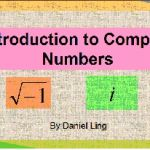## Master the Fundamentals of Vectors

A course to learn the basics of vector algebra
Video Beginner
Gallery
Description
This course is designed specially for students who are: doing college-level mathematics, taking their IGCSE/GCE A level, the IB HL Math examinations or simply anyone who is interested to learn the basics of vectors. At the end of the course, and depending on which exams the students are taking, they will learn most/all of the following: -basic vector operations -ratio theorem and midpoint theorem -scalar (dot) product -length of projection -vector (cross) product -vector equation of a straight line -acute angle between two lines -shortest distance from a point to a line -equations of a plane (scalar-product, Cartesian, parametric form) -distance from a point to a plane -point of intersection between a plane and a line -point of reflection about a plane -acute angle between a plane and a line -angle between two planes -intersection of two planes -intersection of three planes -distance between two planes Along the way, there will be quizzes and practice questions for students to get familiarize with the concepts relating to vectors. There are also several bonus lectures which will further enhance the understanding of the topic. There are a total of 47 lectures, with mostly video presentations.

Pricing:
11.99\$
Level:
Beginner
Duration:
4.5 Stunden On-Demand-Video
Educator:
Ling Meng Kay Daniel
Submitted by:
mkling Author
Reviews
Would you recomment this course to a friend?
Discussion